« Submodular Optimization and Approximation Algorithms

Satoru Iwata

Submodular functions are discrete analogues of convex functions. Examples include cut capacity functions, matroid rank functions, and entropy functions. Submodular functions can be minimized in polynomial time, which provides a fairly general framework of efficiently solvable combinatorial optimization problems. In contrast, the maximization problems are NP-hard and several approximation algorithms have been developed so far. In this lecture, I will review the above results in submodular optimization and present recent approximation algorithms for combinatorial optimization problems described in terms of submodular functions.

Scroll with j/k | | | Size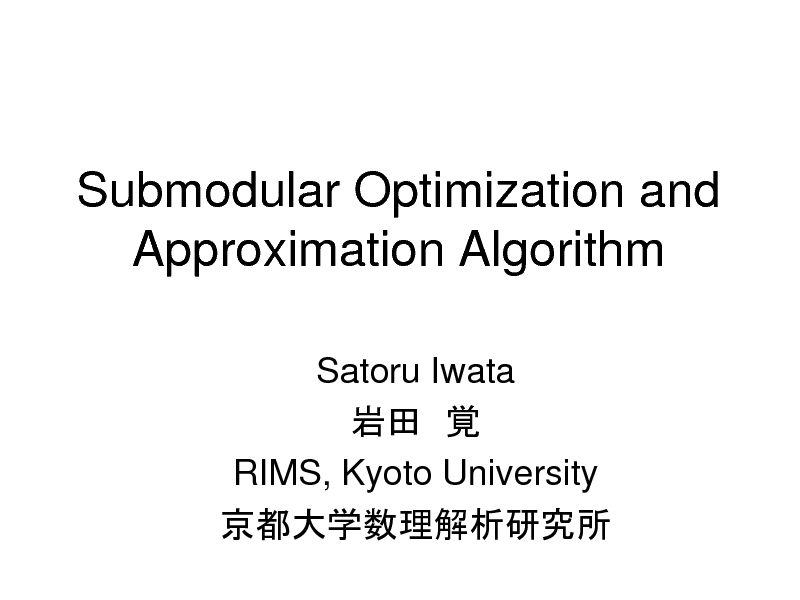Submodular Optimization and Approximation Algorithm
Satoru Iwata RIMS, Kyoto University

1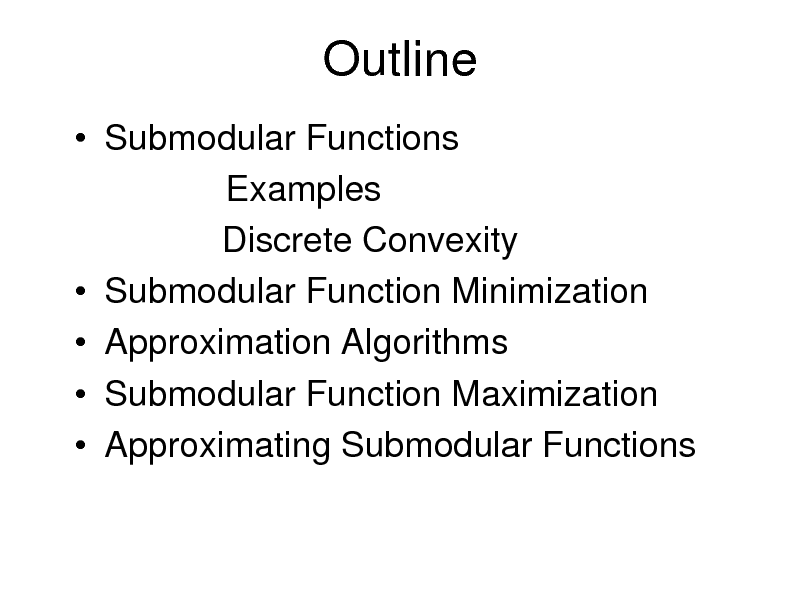Outline
Submodular Functions Examples Discrete Convexity Submodular Function Minimization Approximation Algorithms Submodular Function Maximization Approximating Submodular Functions

2Submodular Functions
V:
Finite Set
V

f :2 R

X , Y V

f ( X ) f (Y ) f ( X Y ) f ( X Y )
Cut Capacity Functions Matroid Rank Functions Entropy Functions

X

Y

V

3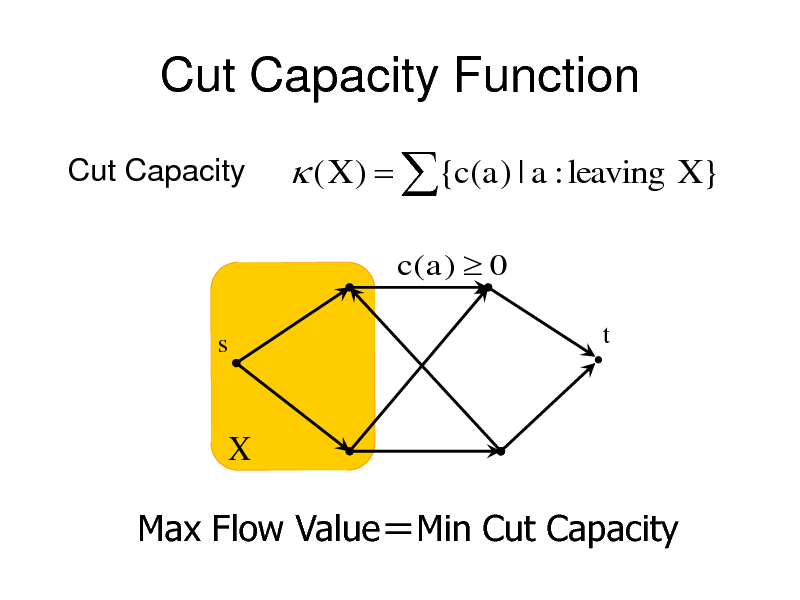Cut Capacity Function
Cut Capacity

( X ) {c(a) | a : leaving X }
c( a ) 0

s

t

X

Max Flow ValueMin Cut Capacity

4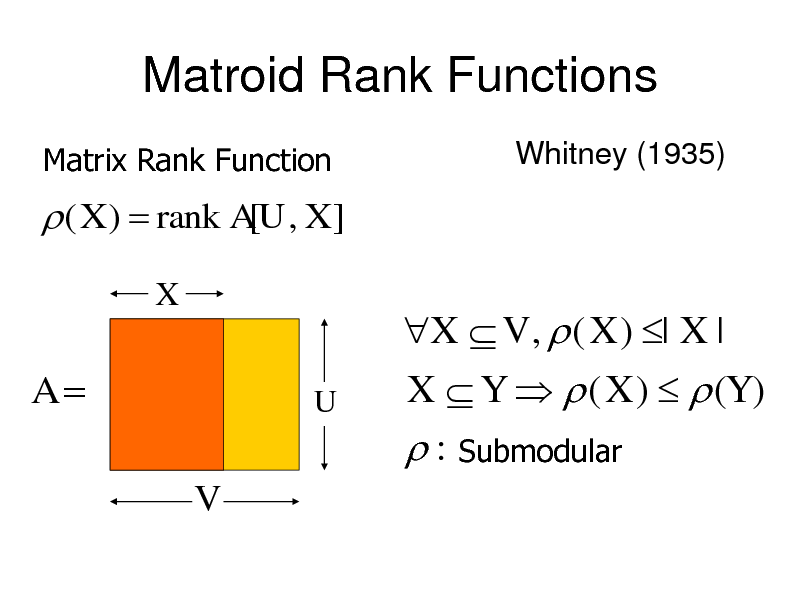Matroid Rank Functions
Matrix Rank Function Whitney (1935)

( X ) rank A[U , X ]
X

A
V

U

X V , ( X ) | X | X Y ( X ) (Y ) : Submodular

5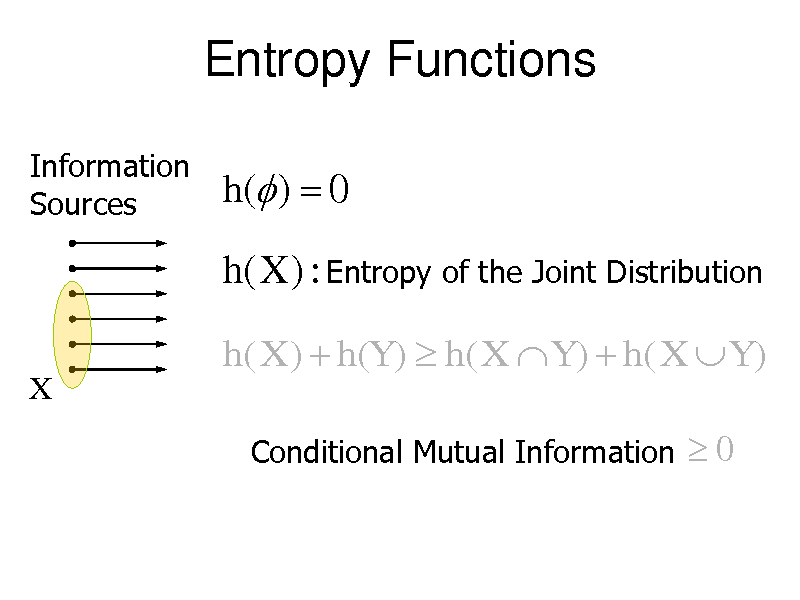Entropy Functions
Information Sources

h( ) 0

h(X ) : Entropy of the Joint Distribution
h( X ) h(Y ) h( X Y ) h( X Y )
X
Conditional Mutual Information

0

6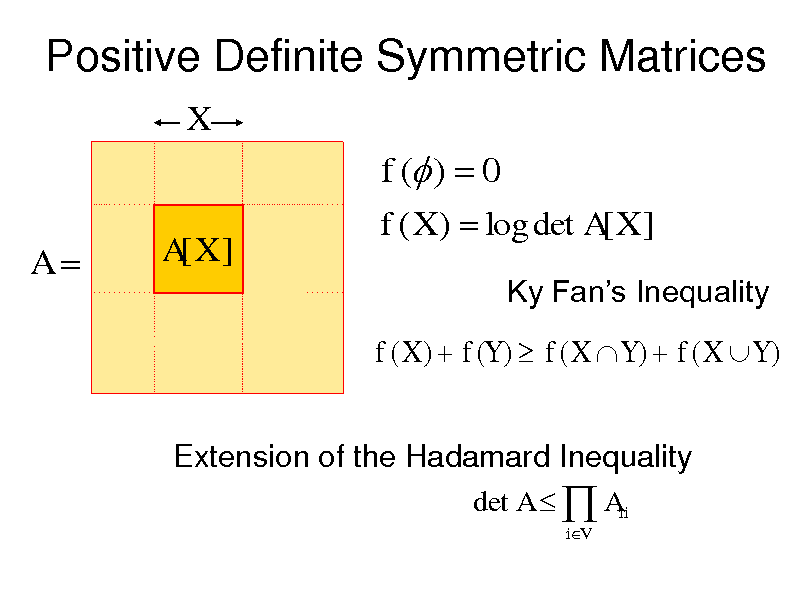Positive Definite Symmetric Matrices
X

f ( ) 0
A
A[X ] f ( X ) log det A[ X ]
Ky Fans Inequality

f ( X ) f (Y ) f ( X Y ) f ( X Y )
det A Aii
iV

7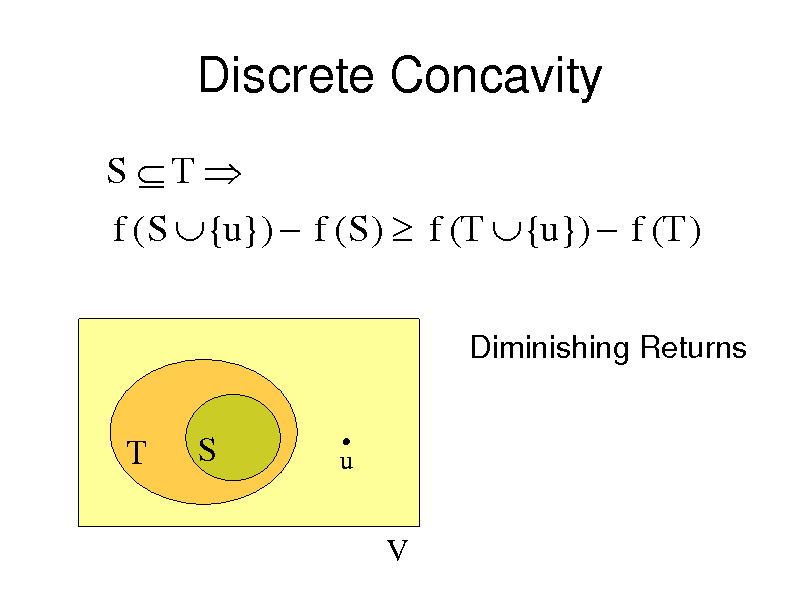Discrete Concavity
S T f ( S {u}) f ( S ) f (T {u}) f (T )
Diminishing Returns

T

S

u

V

8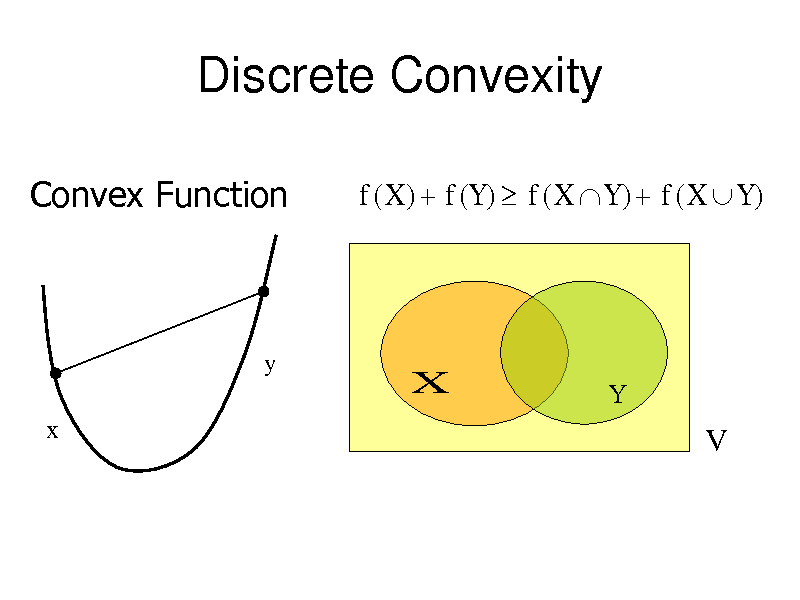Discrete Convexity
Convex Function
f ( X ) f (Y ) f ( X Y ) f ( X Y )

y

X

Y

x

V

9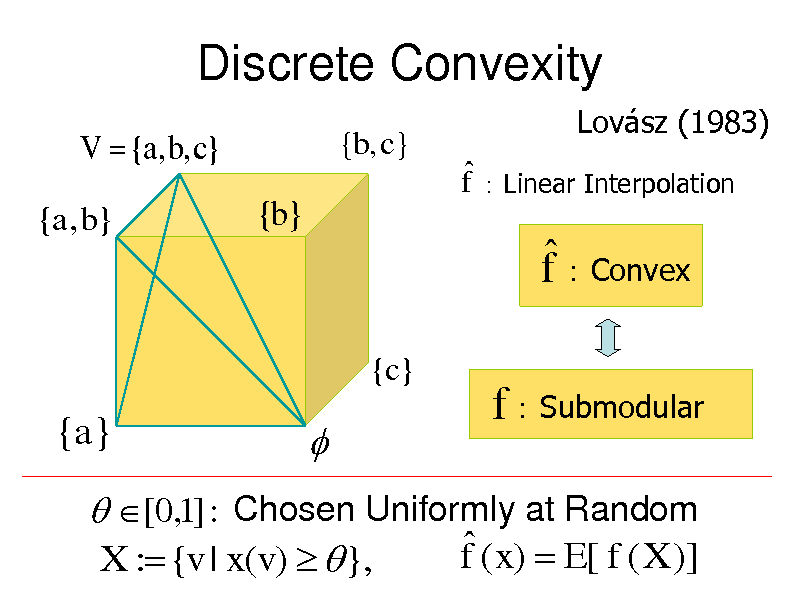Discrete Convexity
V {a, b, c}
{a, b}
{b, c}
Lovsz (1983)

f Linear Interpolation

{b}

f Convex
{c}

{a}

f Submodular

[0,1] : Chosen Uniformly at Random f ( x) E[ f ( X )] X : {v | x(v) },

10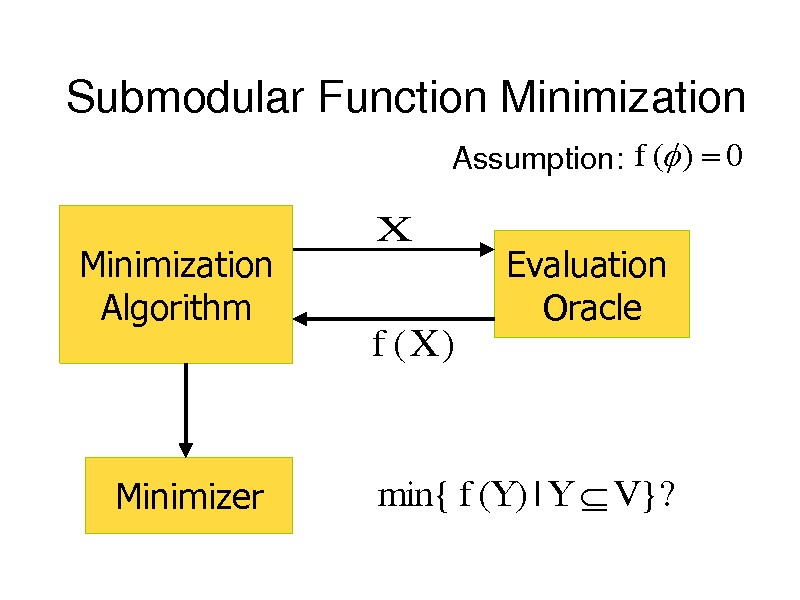Submodular Function Minimization
Assumption: f ( ) 0

Minimization Algorithm

X

Evaluation Oracle

f (X )

Minimizer

min{ f (Y ) | Y V }?

11Submodular Function Minimization
Grtschel, Lovsz, Schrijver (1981, 1988)
Ellipsoid Method

O(n5 log M ) O(n7 log n)

Cunningham (1985)

O( n 7 n 8 )
Schrijver (2000)

Iwata, Fleischer, Fujishige (2000)

: Time for Function Evaluation

M max | f ( X ) |
X V

12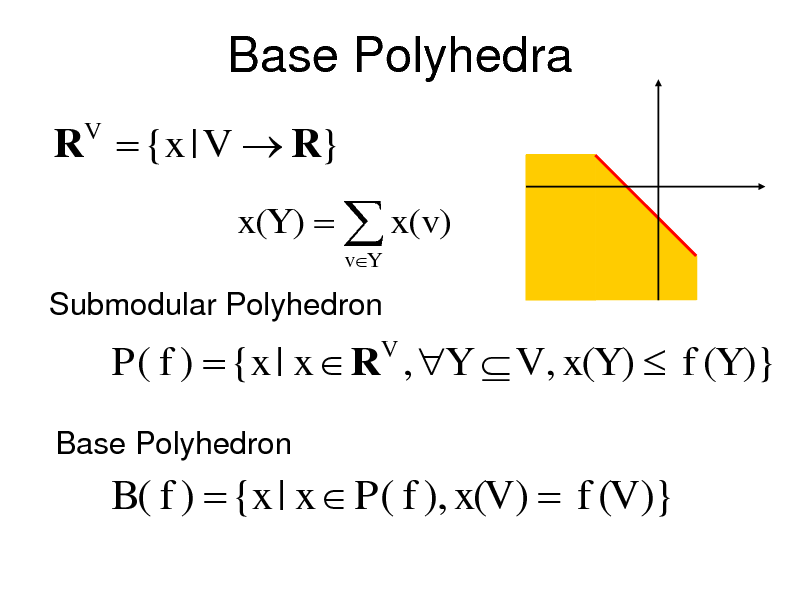Base Polyhedra
R {x | V R}
V

x(Y ) x(v)
vY

Submodular Polyhedron

P( f ) {x | x R , Y V , x(Y ) f (Y )}
V

Base Polyhedron

B( f ) {x | x P( f ), x(V ) f (V )}

13Greedy Algorithm
L(v)

Edmonds (1970) Shapley (1971)

v1

v2

v

vn

y(v) f ( L(v)) f ( L(v) {v})

(v V )

y : Extreme Base
1 1 1 0 1 1 0 y (v1 ) f ( L(v1 )) y (v ) f ( L(v )) 2 2 0 1 y (vn ) f ( L(vn ))

14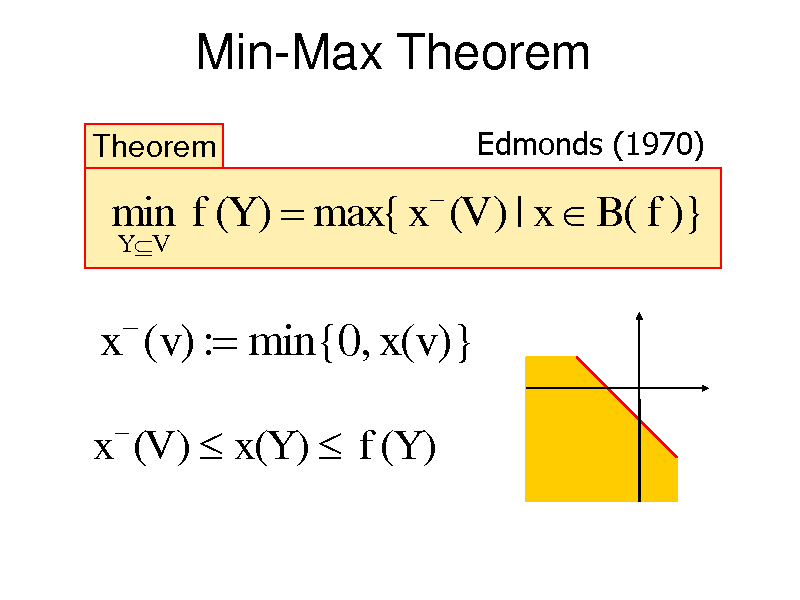Min-Max Theorem
Theorem
Y V

Edmonds (1970)

min f (Y ) max{ x (V ) | x B( f )}

x (v) : min{0, x(v)}
x (V ) x(Y ) f (Y )

15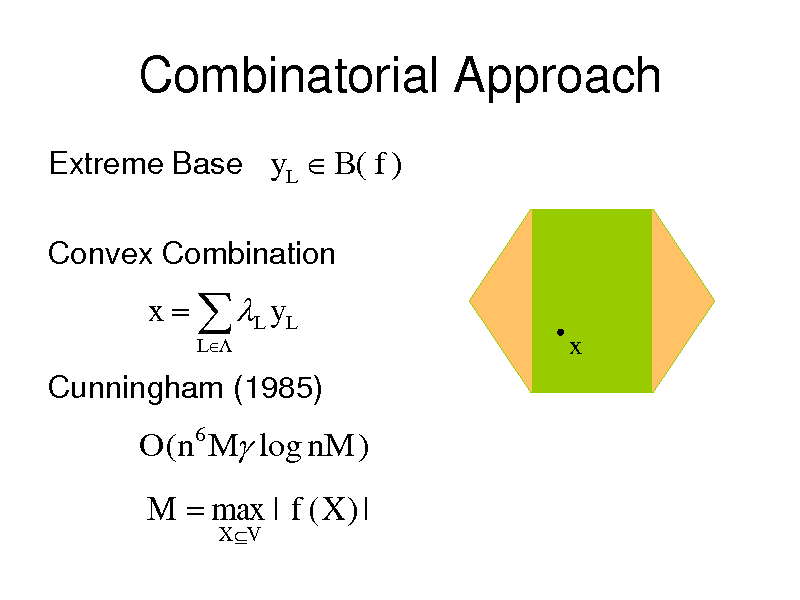Combinatorial Approach
Extreme Base yL B( f )
Convex Combination

x L y L
L

x

Cunningham (1985)

O(n6 M log nM )

M max | f ( X ) |
X V

16Combinatorial Approach
x L y L
L

L

y L : Extreme Base

x(v) 0, v T x(v) 0, v T

T

yL (T ) f (T ), L . x(T ) f (T ).
x (V ) x(T ) f (T )

T : Minimizer

17Submodular Function Minimization
Grtschel, Lovsz, Schrijver (1981, 1988)
Ellipsoid Method

O(n5 log M ) O(n7 log n)

Cunningham (1985)

O( n 7 n 8 )
Schrijver (2000)
Fleischer, Iwata (2000)

Iwata, Fleischer, Fujishige (2000)

Iwata (2002)
Fully Combinatorial

Iwata (2003)

Orlin (2007)

O((n 4 n5 ) log M )
Iwata, Orlin (2009)

O(n5 n6 )

18The Fujishige-Wolfe Algorithm
Minimize x
2

Fujishige (1984)

subject to x B( f )

x : opt.sol.
S : {v | x (v) 0}
T : {v | x (v) 0}

x

Minimal Minimizer Maximal Minimizer

f (S ) f (T ) min f ( X )
X V

19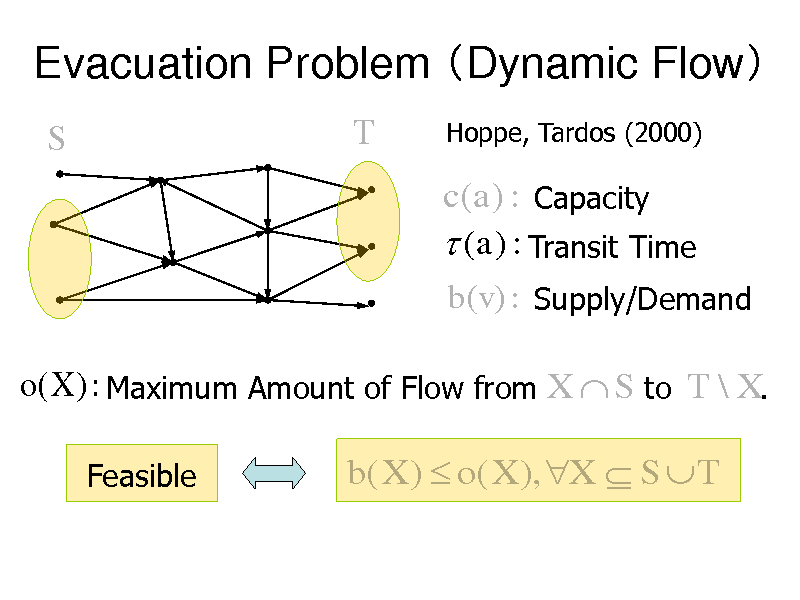Evacuation Problem Dynamic Flow
S
T
Hoppe, Tardos (2000)

c(a) : Capacity (a) : Transit Time
b(v) : Supply/Demand

o(X ) : Maximum Amount of Flow from X S to T \ X.
Feasible

b( X ) o( X ), X S T

20Multiterminal Source Coding
RY

X

Encoder
Encoder

RX

Y

RY

Decoder

H ( X ,Y )
H (Y )

H (Y | X )

Slepian, Wolf (1973)
H(X | Y)
H (X ) H ( X , Y )

RX

21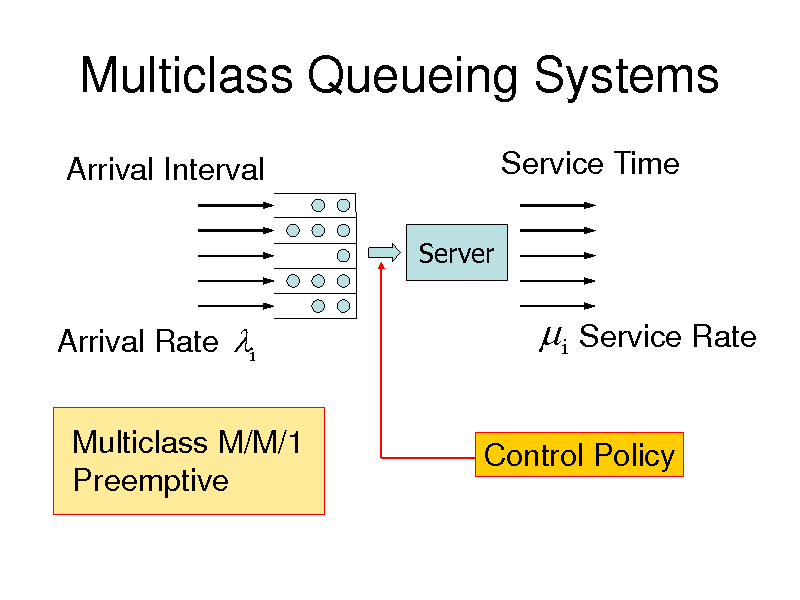Multiclass Queueing Systems
Arrival Interval
Server

Service Time

Arrival Rate i Multiclass M/M/1 Preemptive

i Service Rate
Control Policy

22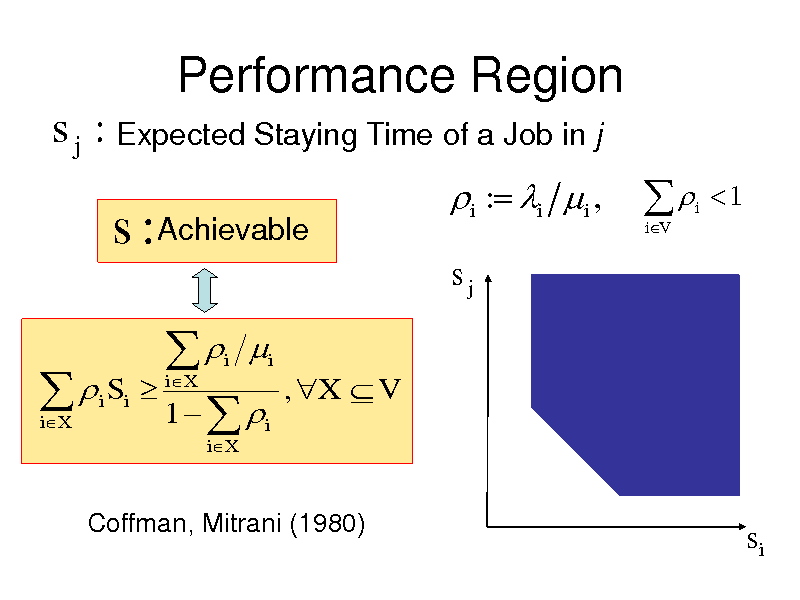Performance Region
s j : Expected Staying Time of a Job in j

s : Achievable
i Si
iX

i : i i ,
sj
RY
s:

iV

i

1

1
iX i iX

i

, X V

i

Coffman, Mitrani (1980)

si

23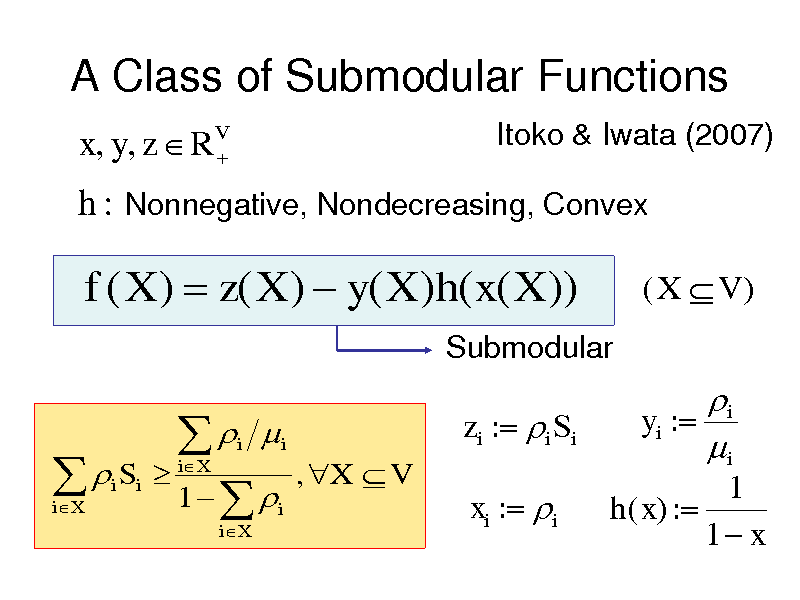A Class of Submodular Functions
x, y, z RV
Itoko & Iwata (2007)

h : Nonnegative, Nondecreasing, Convex

f ( X ) z( X ) y( X )h( x( X ))
Submodular

(X V )

i Si
iX

1
iX i iX

i

zi : i Si
, X V

i yi : i
1 h( x) : 1 x

i

xi : i

24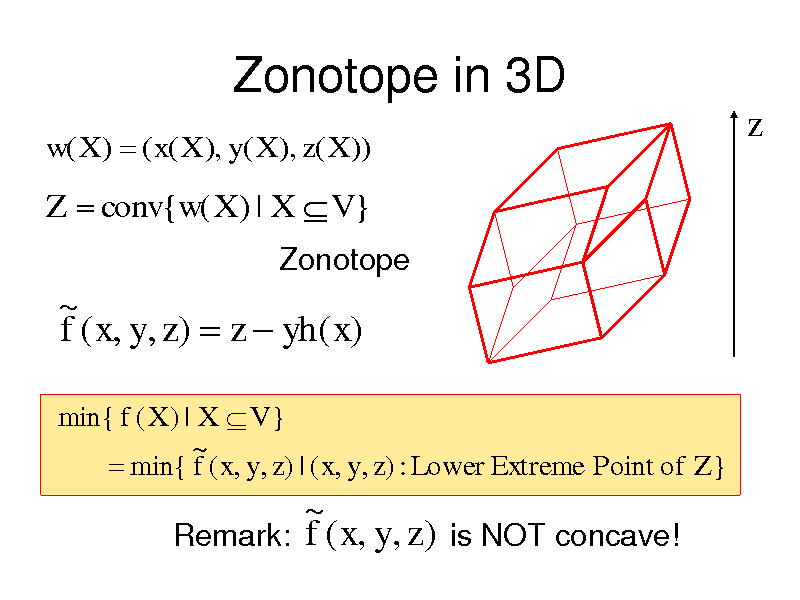Zonotope in 3D
w( X ) ( x( X ), y( X ), z( X ))

z

Z conv{w( X ) | X V }
Zonotope

~ f ( x, y, z ) z yh( x)
min{ f ( X ) | X V } ~ min{ f ( x, y, z ) | ( x, y, z ) : Lower Extreme Point of Z }

~ Remark: f ( x, y, z ) is NOT concave!

25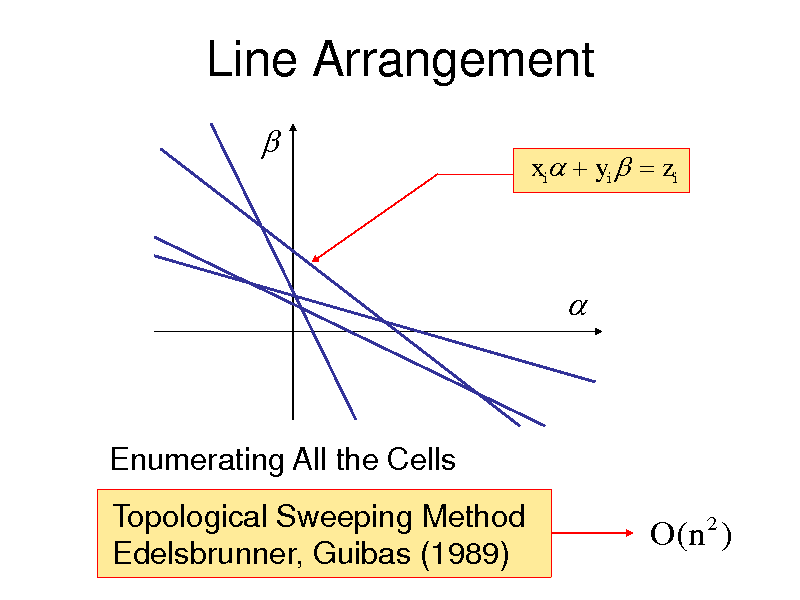Line Arrangement

xi yi zi

Enumerating All the Cells Topological Sweeping Method Edelsbrunner, Guibas (1989)

O( n 2 )

26Symmetric Submodular Functions
f :2 R
V

Symmetric

f ( X ) f (V \ X ),

X V .

Symmetric Submodular Function Minimization

min{ f ( X ) | X V }?
O(n3 ) Queyranne (1998)
Minimum Cut Algorithm by MA-ordering Nagamochi & Ibaraki (1992) Minimum Degree Ordering Nagamochi (2007)

27Submodular Partition
Minimize subject to

f (V )
i 1 i

k

Greedy Split

V V1 Vk
Vi V j (i j )

f : Monotone or Symmetric

2(1 1 k )-Approximation
Zhao, Nagamochi, Ibaraki (2005)

28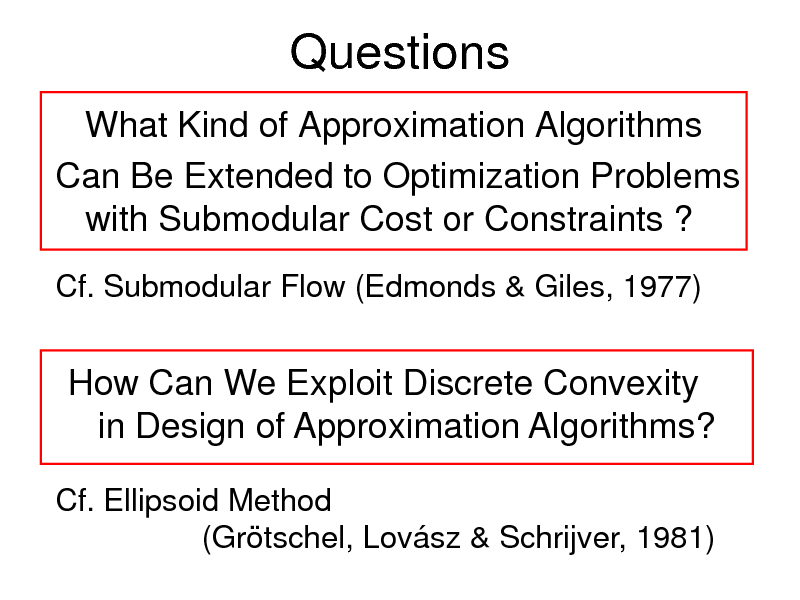Questions
What Kind of Approximation Algorithms Can Be Extended to Optimization Problems with Submodular Cost or Constraints ?
Cf. Submodular Flow (Edmonds & Giles, 1977)

How Can We Exploit Discrete Convexity in Design of Approximation Algorithms?
Cf. Ellipsoid Method (Grtschel, Lovsz & Schrijver, 1981)

29Submodular Vertex Cover
Graph G (V , E )
Submodular Function
f : 2V R

Find a Vertex Cover S V Minimizing f (S ) 2-Approximation Algorithm
Goel, Karande, Tripathi, Wang (FOCS 2009) Iwata & Nagano (FOCS 2009)

30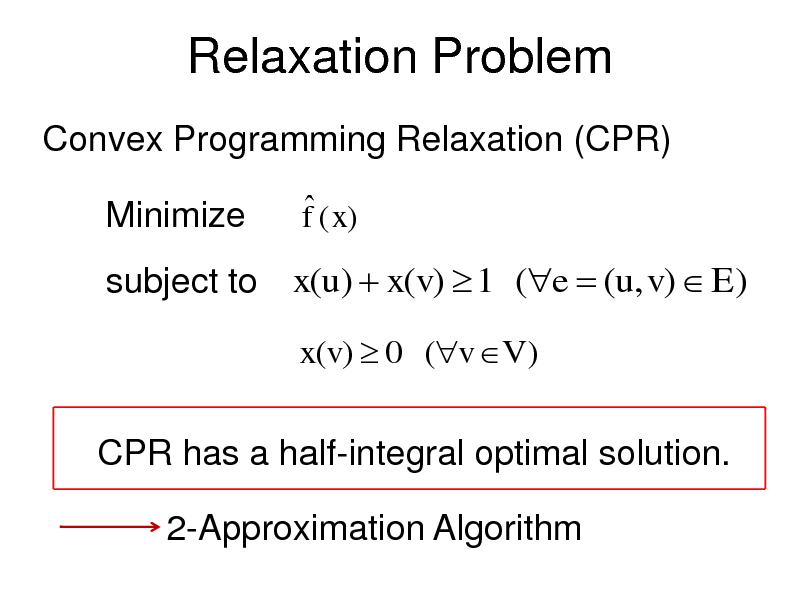Relaxation Problem
Convex Programming Relaxation (CPR)

Minimize

f ( x)

subject to x(u) x(v) 1 (e (u, v) E)
x(v) 0 (v V )

CPR has a half-integral optimal solution. 2-Approximation Algorithm

31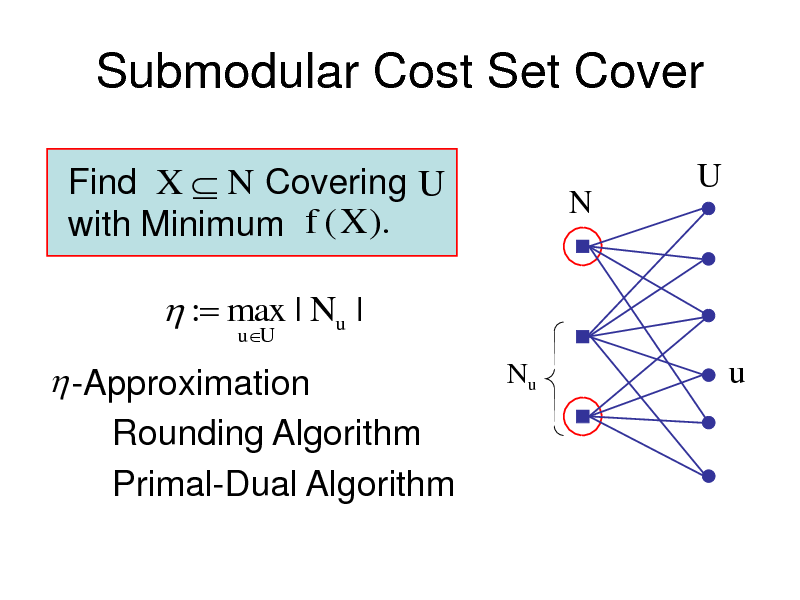Submodular Cost Set Cover
Find X N Covering U with Minimum f (X ).
N

U

: max | Nu |
uU

-Approximation Rounding Algorithm Primal-Dual Algorithm

Nu

u

32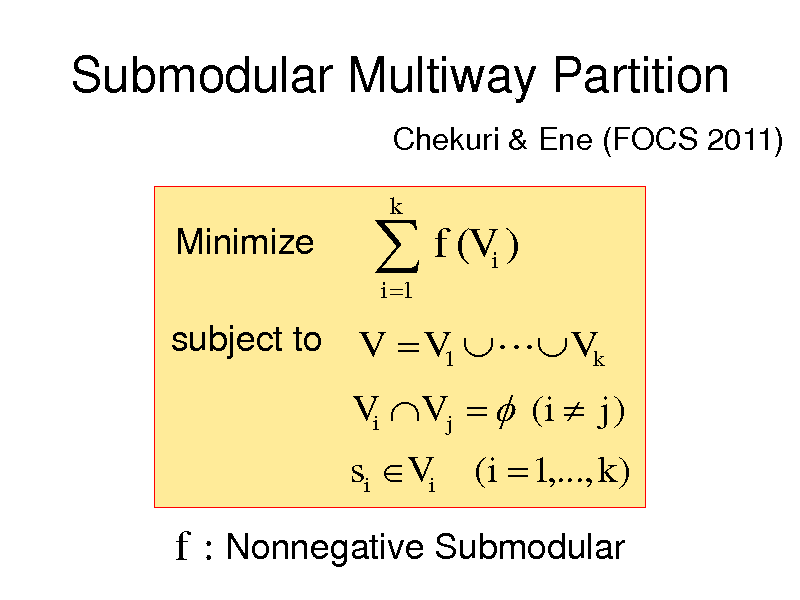Submodular Multiway Partition
Chekuri & Ene (FOCS 2011)

Minimize

f (V )
i 1 i

k

subject to V V1 Vk

Vi V j (i j )

si Vi

(i 1,..., k )

f : Nonnegative Submodular

33Relaxation Problem
Convex Programming Relaxation

Minimize
subject to

i 1 k

k

f ( xi )
i

x (v ) 1
i 1

(v V )

xi (si ) 1 (i 1,..., k )
xi (v) 0
Ellipsoid Method

(i 1,..., k , v V )

xi : Optimal Solution

34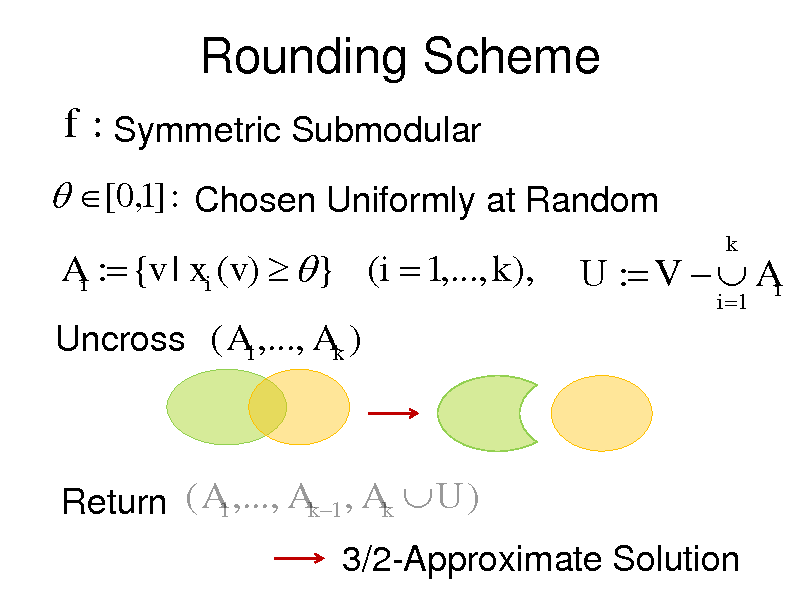Rounding Scheme
f : Symmetric Submodular
[0,1] : Chosen Uniformly at Random
Ai : {v | xi (v) } (i 1,..., k ),
Uncross ( A1 ,..., Ak )

U : V Ai
i 1

k

Return ( A1 ,..., Ak 1 , Ak U ) 3/2-Approximate Solution

35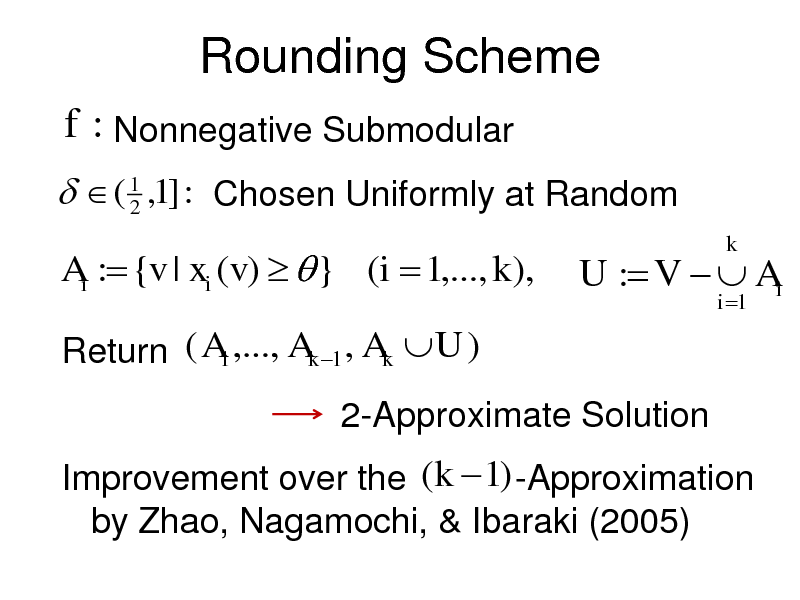Rounding Scheme
f : Nonnegative Submodular
( 1 ,1] : Chosen Uniformly at Random 2
Ai : {v | xi (v) } (i 1,..., k ),
Return ( A1 ,..., Ak 1 , Ak U )
2-Approximate Solution Improvement over the (k 1) -Approximation by Zhao, Nagamochi, & Ibaraki (2005)

U : V Ai
i 1

k

36Submodular Function Maximization
Monotone Submodular Functions
Nemhauser, Wolsey, Fisher (1978) Cardinality Constraint (1-1/e)-Approximation

Calinescu, Chekuri, Pl, Vondrk (IPCO 2007) Vondrk (STOC 2008) Filmes and Ward (FOCS 2012) Matroid Constraint (1-1/e)-Approximation

37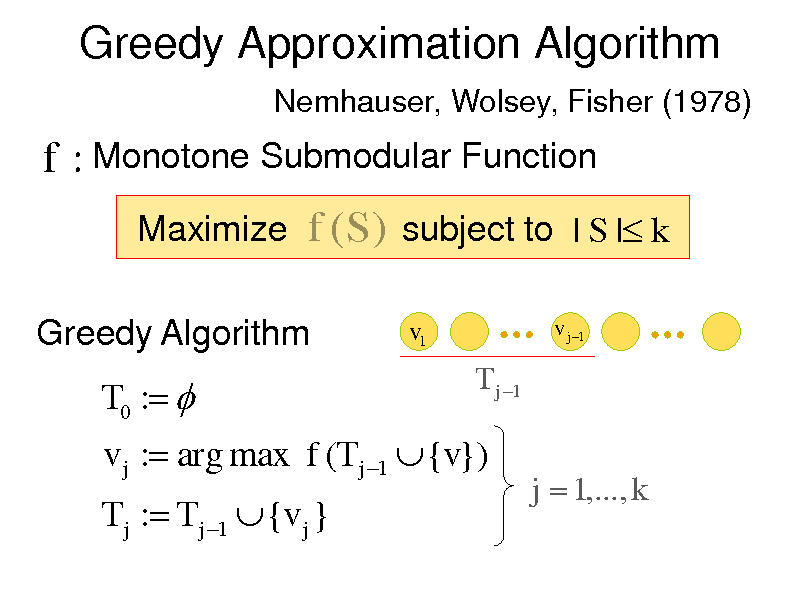Greedy Approximation Algorithm
Nemhauser, Wolsey, Fisher (1978)

f : Monotone Submodular Function
Maximize f (S ) subject to | S | k Greedy Algorithm
v1
v j 1

T0 : T j : T j 1 {v j }

T j 1

v j : arg max f (T j 1 {v})

j 1,..., k

38Greedy Approximation Algorithm
S : Optimal Solution : f (S )
*
*

k j i ( j 1,..., k )
i 1

j 1

j : f (T j ) f (T j 1 )

f (Tk ) i
i 1

k

) f ( S ) f ( S T j 1 )
* *

f (T j 1 )

uS * \T j 1

f (T

j 1

{u}) f (T j 1 )
j 1

f (T j 1 ) k j

i 1

i

39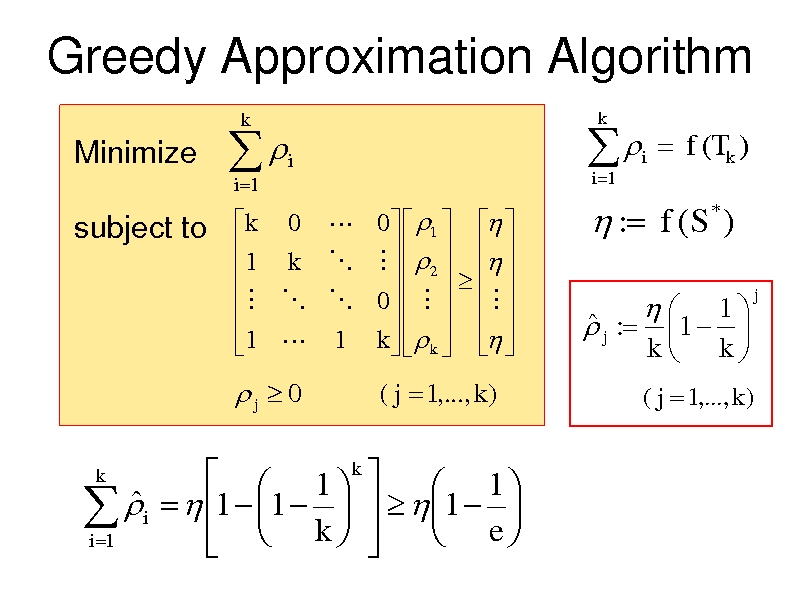Greedy Approximation Algorithm
Minimize

i 1

k

i

i 1

k

i

f (Tk )
*

subject to

k 0 0 1 1 k 2 0 1 1 k k

: f (S )
j :

1 1 k k

j

j 0
k

( j 1,..., k )

( j 1,..., k )

1 k 1 i 1 1 k 1 e i 1

40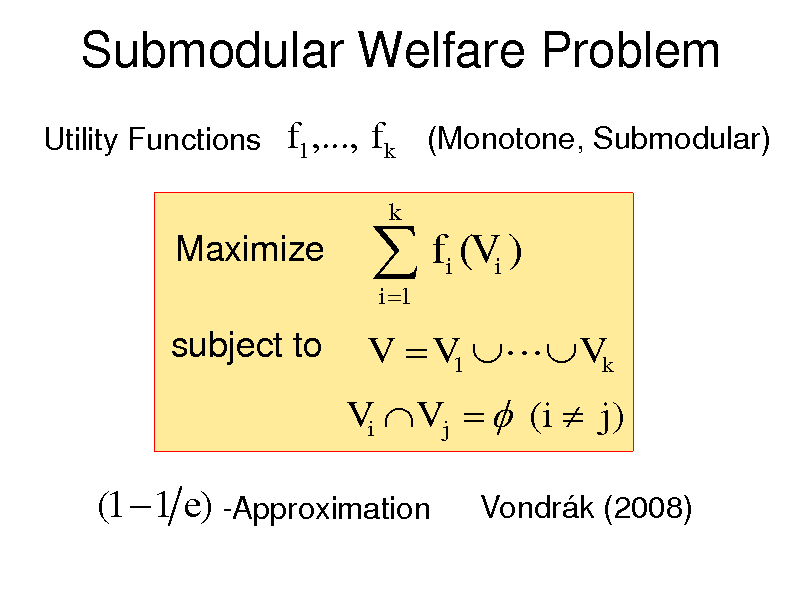Submodular Welfare Problem
Utility Functions

f1 ,..., f k (Monotone, Submodular)

Maximize subject to

f (V )
i 1 i i

k

V V1 Vk
Vi V j (i j )

(1 1 e) -Approximation

Vondrk (2008)

41Submodular Function Maximization
Nonnegative Submodular Functions
Feige, Mirrokni, Vondrk (FOCS 2007) 2/5-Approximation Lee, Mirrokni, Nagarajan, Sviridenko (STOC 2009) 1/4-Approximation (Matroid Constraint) 1/5-Approximation (Knapsack Constraints) Buchbinder, Feldman, Naor, Schwartz (FOCS 2012) 1/2-Approximation

42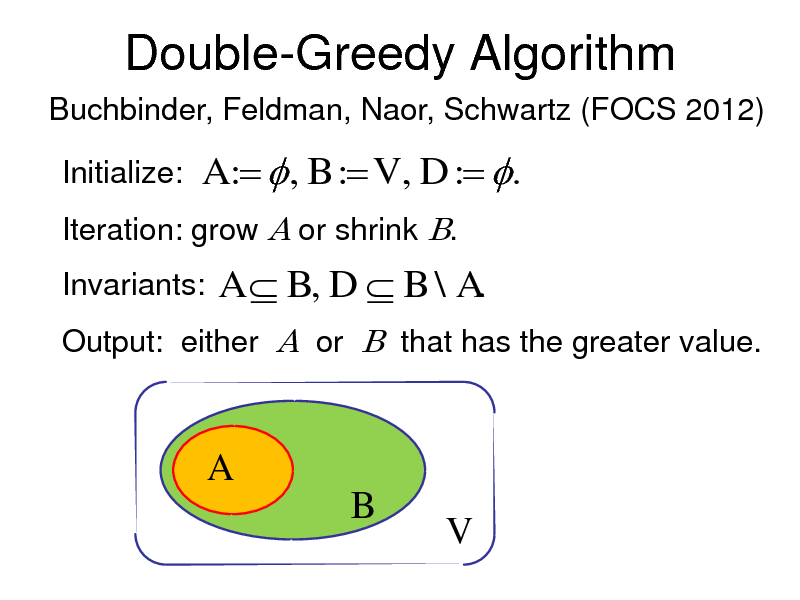Double-Greedy Algorithm
Buchbinder, Feldman, Naor, Schwartz (FOCS 2012)

Initialize:

A : , B : V , D : . A B, D B \ A.

Iteration: grow A or shrink B. Invariants: Output: either A or B that has the greater value.

A B
V

43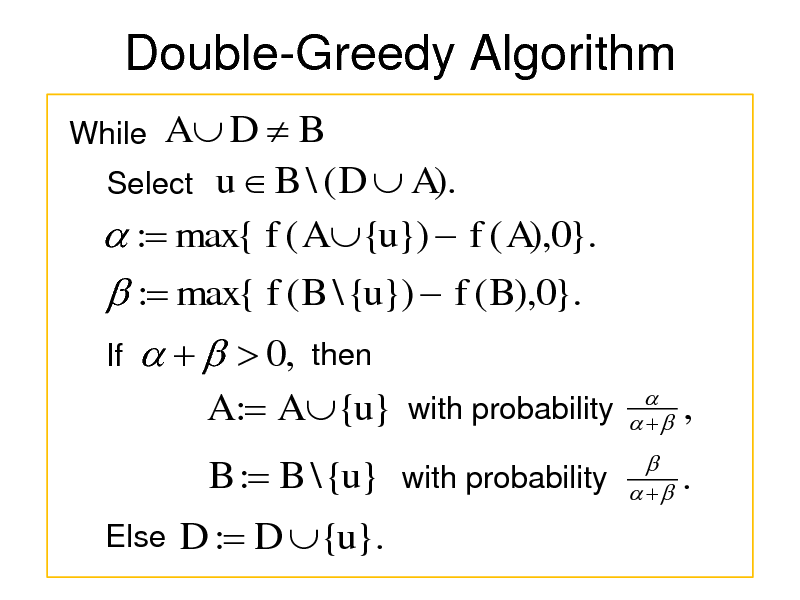Double-Greedy Algorithm
While

A D B Select u B \ ( D A). : max{ f ( A {u}) f ( A),0}. : max{ f ( B \ {u}) f ( B),0}.
If

0,

then

A : A {u} with probability B : B \ {u} with probability
Else

,

.

D : D {u}.

44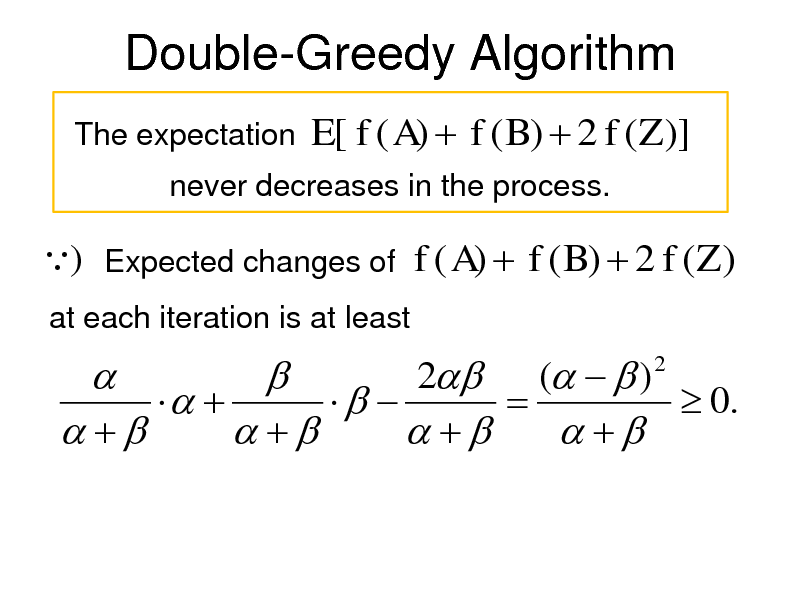Double-Greedy Algorithm
The expectation

E[ f ( A) f ( B) 2 f (Z )]

never decreases in the process.

) Expected changes of f ( A) f ( B) 2 f (Z )
at each iteration is at least

2 ( ) 0.
2

45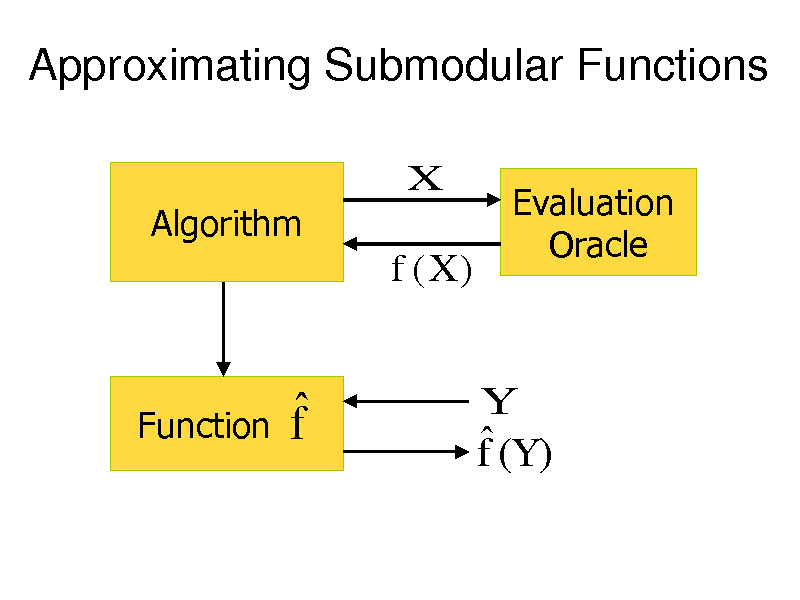Approximating Submodular Functions
X
Algorithm

f (X )

Evaluation Oracle

Function

f

f (Y )

Y

46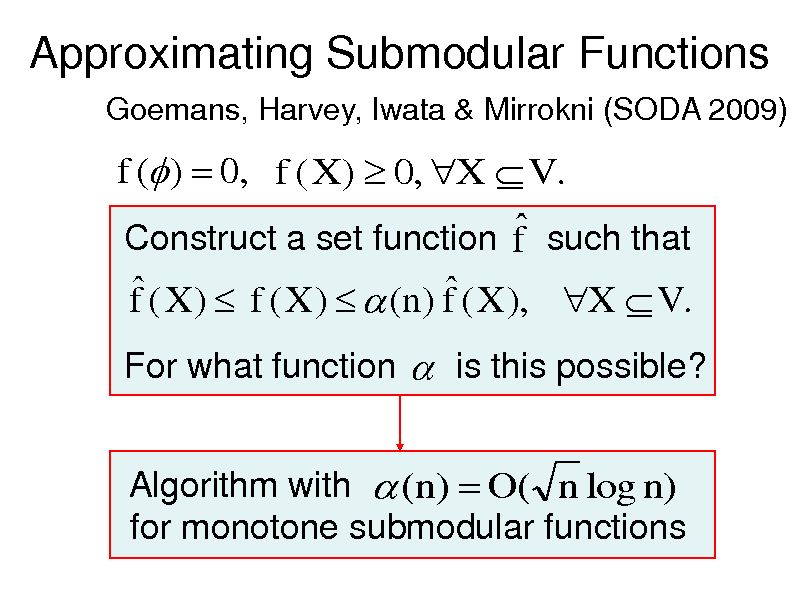Approximating Submodular Functions
Goemans, Harvey, Iwata & Mirrokni (SODA 2009)

f ( ) 0, f ( X ) 0, X V . Construct a set function f such that
f ( X ) f ( X ) (n) f ( X ), X V .
For what function is this possible? Algorithm with (n) O( n log n) for monotone submodular functions

47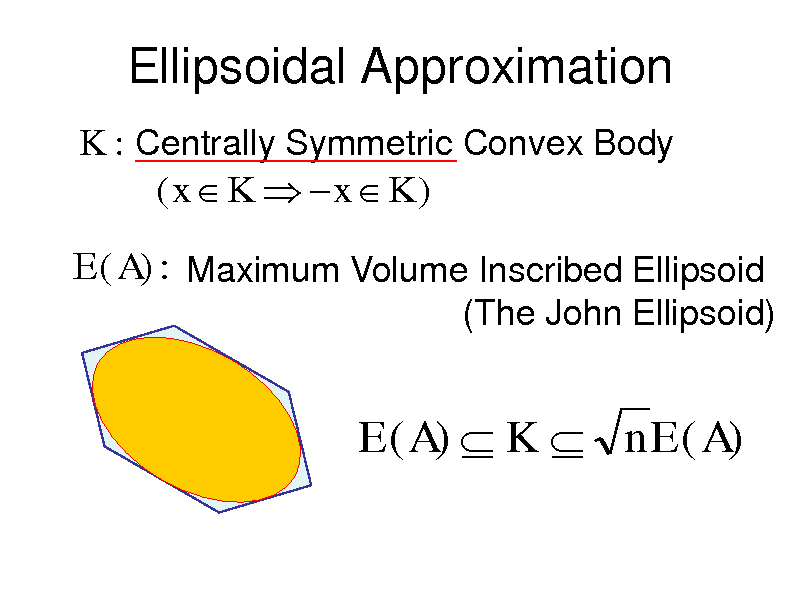Ellipsoidal Approximation
K : Centrally Symmetric Convex Body (x K x K )

E (A) : Maximum Volume Inscribed Ellipsoid
(The John Ellipsoid)

E ( A) K n E ( A)

48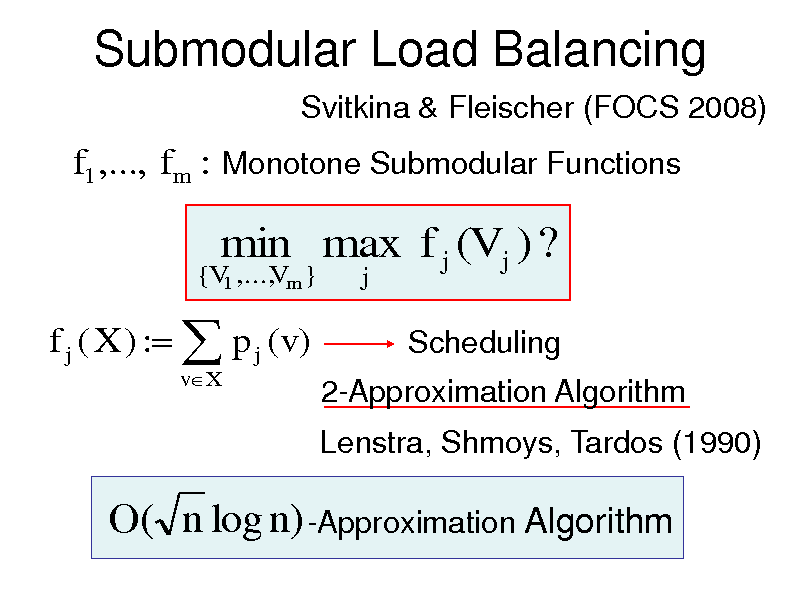Svitkina & Fleischer (FOCS 2008)

f1 ,..., f m : Monotone Submodular Functions
{V1 ,..., m } V

min max f j (V j ) ?
j

f j ( X ) : p j (v)
vX

Scheduling 2-Approximation Algorithm Lenstra, Shmoys, Tardos (1990)

O( n log n) -Approximation Algorithm

49Learning Submodular Functions
Balcan & Harvey (STOC 2010)

PMAC-learning

Construct a set function f such that f ( X ) f ( X ) (n) f ( X )
holds for most (1 fraction) of X . For what function is this possible with probability 1 in poly(n,1 / ,1 / ) time?

50Summary
Submodular Functions Arise Everywhere. Discrete Analogue of Convexity. Combinatorial Algorithms for Minimization. Exploit Special Structures of Problems. Approx. Algorithms by Discrete Convexity Approx. Algorithms for Maximization Learning Submodular Functions

51